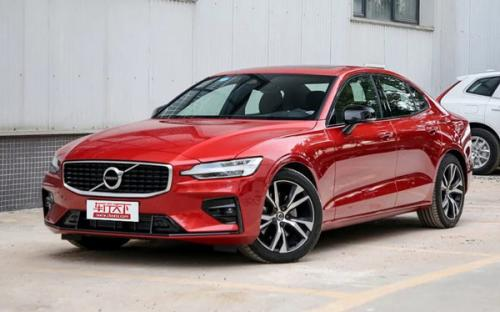### 沃尔沃亚太 沃尔沃S602020款最低售价：28.70 万元起

4761×1850×1437(mm)(mm)(mm)##### 配置亮点：
• 胎压监测装置

• ISOFIX儿童座椅接口

• 车身稳定控制(ESC/ESP/DSC等)

• 电动天窗

• 定速巡航

• 后倒车雷达

• 真皮座椅

• GPS导航系统

• 氙气大灯

• 后视镜加热

• 提交
2020款 T5 智雅运动版 (147张)

沃尔沃亚太 沃尔沃S60 绕车实拍• 沃尔沃亚太 沃尔沃S60 在售车型

排量 车型 厂商指导价 本地最低报价 购车工具
2.0TT
T3 智行豪华版 8挡手自一体
28.69万
28.69万

T4 智逸豪华版 8挡手自一体
30.29万
30.29万

T4 智远豪华版 8挡手自一体
33.58万
T4 智远运动版 8挡手自一体
33.58万
T5 智雅运动版 8挡手自一体
37.99万
37.99万

沃尔沃亚太 沃尔沃S60 经销商

查看更多 >>

### 沃尔沃亚太 沃尔沃S60 动力加速

沃尔沃S60 0-100公里加速时间分布在 6.5-8.9秒 属于 高性能级

动力级别 加速时间 车型
运动级(2款)
8.9s
T3 智行豪华版
7.7s
T4 智逸豪华版T4 智远豪华版T4 智远运动版
高性能级(1款)
6.5s
T5 智雅运动版

沃尔沃亚太 沃尔沃S60 视频

沃尔沃亚太 沃尔沃S60 新闻资讯

# 沃尔沃S60面对奥迪A4L 看看到底香在哪里？

试驾评测 超过8463次关注

“亚太沃尔沃”的国产S60换代车型终于要在今年年底正式上市。新一代的S60车型则完全切换到沃尔沃自己的全新SPA模块化平台。独立前悬架从麦弗逊结构变成了同级豪华车...

# 为健康而选择,向佐夫妇、黄磊夫妇罕见同框

国产新车 超过12403次关注

以“为爱选择”为主题的沃尔沃全新S60上市发布会,非常让人触动的地方,是向佐和黄磊两对夫妇的真情告白,简直是一部为爱选择健康座驾的教科书。

# 百公里加速仅需4.6秒沃尔沃变速箱助攻

试驾评测 超过11311次关注

12月12日,沃尔沃汽车在上海市黄埔区中国船舶馆举行上市发布会,来自沃尔沃汽车大庆工厂的全新S60轿车正式向国内市场开启销售。

# 全新沃尔沃S60

即将上市 超过14670次关注

沃尔沃全新S6将于12月12日正式上市,共推出7款车型,分别为T3智行豪华版、T4智逸豪华版、T4智远豪华版、T4智远运动版、T5智雅运动版、T8E驱混动四驱智逸豪华版和T8E驱...

猜你喜欢

﻿
• 快速找车
• 选择品牌
• 选择品牌
• A  奥迪
• A  阿斯顿·马丁
• A  阿尔法·罗密欧
• B  宝沃
• B  布加迪
• B  巴博斯
• B  保时捷
• B  宾利
• B  奔驰
• B  宝马
• B  本田
• B  别克
• B  标致
• B  比亚迪
• B  宝骏
• B  北汽制造
• B  北汽新能源
• B  北汽幻速
• B  北汽威旺
• B  北京汽车
• B  奔腾
• B  北汽绅宝
• C  长安
• C  长安商用
• C  长城
• C  昌河
• D  大众
• D  道奇
• D  DS
• D  东南
• D  东风风神
• D  东风风行
• D  东风小康
• D  东风风度
• D  东风
• F  福特
• F  丰田
• F  菲亚特
• F  法拉利
• F  福田
• F  福迪
• F  福汽启腾
• G  观致
• G  广汽传祺
• G  广汽吉奥
• G  GMC
• H  红旗
• H  汉腾汽车
• H  哈弗
• H  哈飞
• H  海格
• H  海马
• H  华颂
• H  黄海
• H  华泰
• H  恒天
• J  吉利汽车
• J  捷豹
• J  Jeep
• J  江淮
• J  江铃
• J  金杯
• J  九龙
• J  金旅
• K  凯翼
• K  凯迪拉克
• K  克莱斯勒
• K  科尼塞克
• K  卡威
• K  开瑞
• L  路虎
• L  林肯
• L  劳斯莱斯
• L  兰博基尼
• L  雷克萨斯
• L  铃木
• L  雷诺
• L  理念
• L  力帆
• L  莲花汽车
• L  猎豹
• L  路特斯
• L  陆风
• M  马自达
• M  MG
• M  MINI
• M  玛莎拉蒂
• M  摩根
• M  迈凯轮
• N  纳智捷
• O  欧宝
• O  讴歌
• O  欧朗
• Q  奇瑞
• Q  起亚
• Q  启辰
• R  日产
• R  荣威
• R  瑞麒
• S  三菱
• S  斯威汽车
• S  萨博
• S  smart
• S  斯柯达
• S  斯巴鲁
• S  思铭
• S  双龙
• S  上汽大通
• S  双环
• T  特斯拉
• T  腾势
• W  沃尔沃
• W  五菱汽车
• W  五十铃
• W  威兹曼
• W  威麟
• X  现代
• X  雪佛兰
• X  雪铁龙
• X  西雅特
• Y  一汽
• Y  英菲尼迪
• Y  英致
• Y  依维柯
• Y  野马汽车
• Y  永源
• Z  众泰
• Z  中华
• Z  中兴
• Z  知豆
• 选择车系
• 选择车系
• 车型对比
• 选择品牌
• 选择品牌
• A  奥迪
• A  阿斯顿·马丁
• A  阿尔法·罗密欧
• B  宝沃
• B  布加迪
• B  巴博斯
• B  保时捷
• B  宾利
• B  奔驰
• B  宝马
• B  本田
• B  别克
• B  标致
• B  比亚迪
• B  宝骏
• B  北汽制造
• B  北汽新能源
• B  北汽幻速
• B  北汽威旺
• B  北京汽车
• B  奔腾
• B  北汽绅宝
• C  长安
• C  长安商用
• C  长城
• C  昌河
• D  大众
• D  道奇
• D  DS
• D  东南
• D  东风风神
• D  东风风行
• D  东风小康
• D  东风风度
• D  东风
• F  福特
• F  丰田
• F  菲亚特
• F  法拉利
• F  福田
• F  福迪
• F  福汽启腾
• G  观致
• G  广汽传祺
• G  广汽吉奥
• G  GMC
• H  红旗
• H  汉腾汽车
• H  哈弗
• H  哈飞
• H  海格
• H  海马
• H  华颂
• H  黄海
• H  华泰
• H  恒天
• J  吉利汽车
• J  捷豹
• J  Jeep
• J  江淮
• J  江铃
• J  金杯
• J  九龙
• J  金旅
• K  凯翼
• K  凯迪拉克
• K  克莱斯勒
• K  科尼塞克
• K  卡威
• K  开瑞
• L  路虎
• L  林肯
• L  劳斯莱斯
• L  兰博基尼
• L  雷克萨斯
• L  铃木
• L  雷诺
• L  理念
• L  力帆
• L  莲花汽车
• L  猎豹
• L  路特斯
• L  陆风
• M  马自达
• M  MG
• M  MINI
• M  玛莎拉蒂
• M  摩根
• M  迈凯轮
• N  纳智捷
• O  欧宝
• O  讴歌
• O  欧朗
• Q  奇瑞
• Q  起亚
• Q  启辰
• R  日产
• R  荣威
• R  瑞麒
• S  三菱
• S  斯威汽车
• S  萨博
• S  smart
• S  斯柯达
• S  斯巴鲁
• S  思铭
• S  双龙
• S  上汽大通
• S  双环
• T  特斯拉
• T  腾势
• W  沃尔沃
• W  五菱汽车
• W  五十铃
• W  威兹曼
• W  威麟
• X  现代
• X  雪佛兰
• X  雪铁龙
• X  西雅特
• Y  一汽
• Y  英菲尼迪
• Y  英致
• Y  依维柯
• Y  野马汽车
• Y  永源
• Z  众泰
• Z  中华
• Z  中兴
• Z  知豆
• 选择车系
• 选择车系
• 选择车型
• 选择车型
• 意见反馈